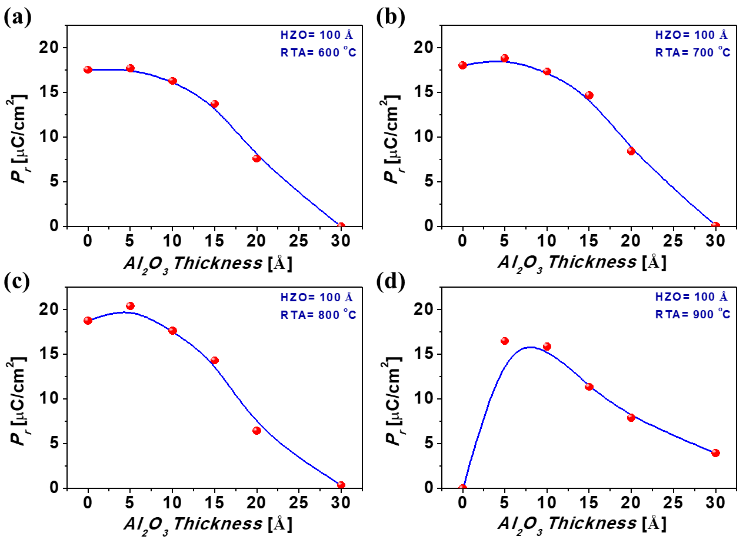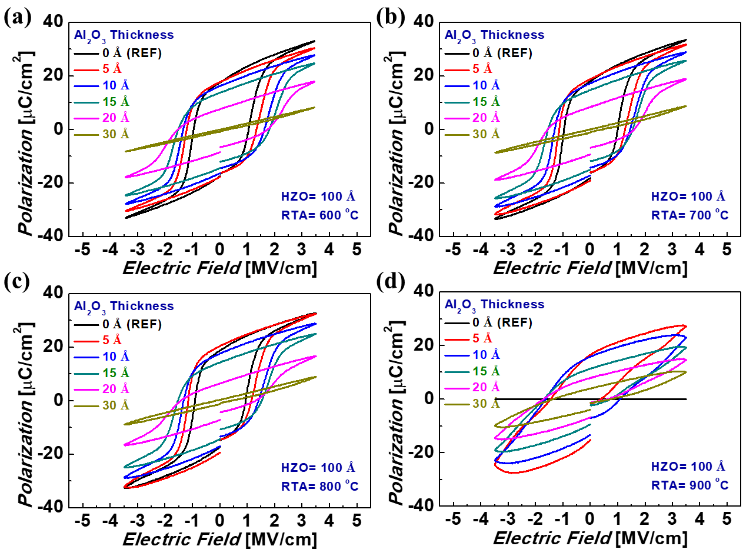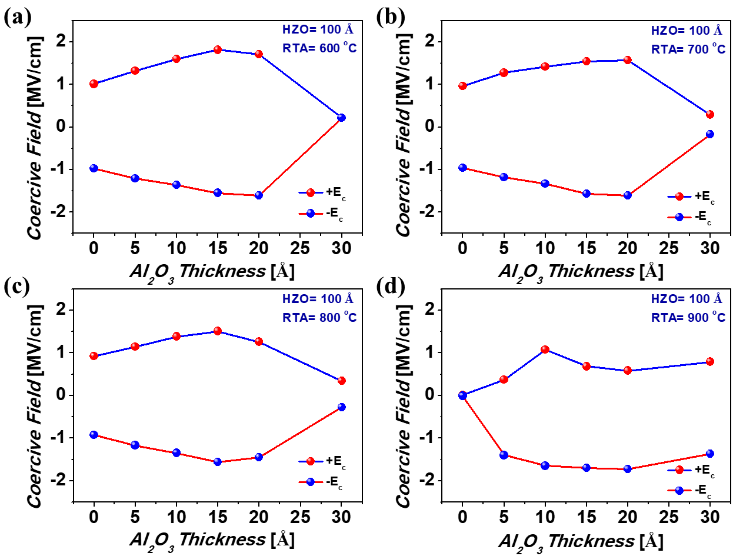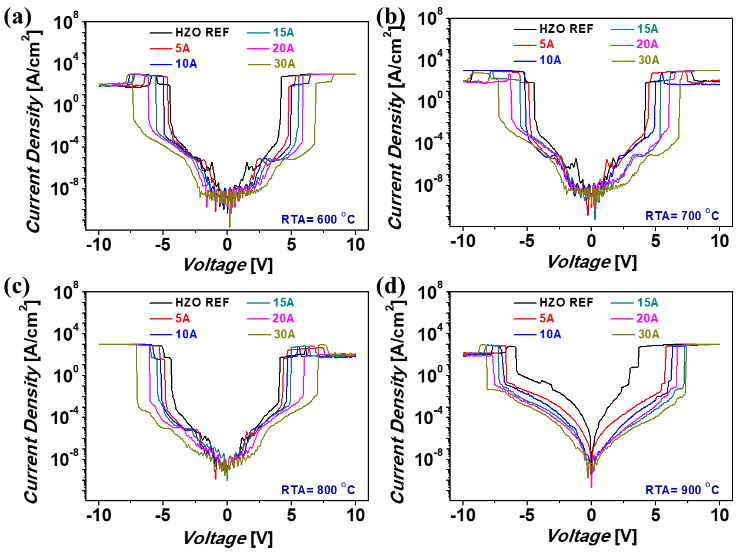Mobile QR Code1. (KAIST - School of Electrical Engineering, Yuseonggu, Daehakro Daejeon 34141, Korea)

Hf$_{\mathbf{0.5}}$Zr$_{\mathbf{0.5}}$O$_{\mathbf{2 }}$(HZO), DE/FE stack remanent polarization, leakage current, thermal stability

## I. INTRODUCTION

Ferroelectricity in undoped and doped HfO$_{2}$ has been exploited substantially in recent years due to its abundant advantages such as wide bang gap, excellent scalability, admirable endurance, reliability and most importantly its adaptability with the current CMOS technology (1-10).

Especially, ferroelectric HfO$_{2}$ based materials have been studied widely as gate for FET based ferroelectric non-volatile memory applications to achieve steep-slope switching by utilizing its negative capacitance property (11-17). In such devices, a ferroelectric (FE) and dielectric (DE) bilayer stack is usually applied as the gate stack. Apart from non-volatile memory applications, the bilayer stack of DE/FE materials has also been explored for capacitance enhancement to attain higher energy storage and capacitance density in electrostatic storage and DRAM applications respectively (18-22). Considering the tremendous attention received by the DE/FE stack, it is very important to understand the influence of the DE layer on the ferroelectric properties of the FE materials in such stacks. In case of Metal Ferroelectric Metal (MFM) capacitor, the charges due to the spontaneous polarization of the FE material are screened by the metal electrode. On the contrary, insertion of a dielectric layer in between the ferroelectric layer and the metal electrode results in unscreened charges which in turn leads to an internal and depolarization field across the DE and FE layer respectively thereby effecting the ferroelectric properties of the FE layer (23). Recently, Al$_{2}$O$_{3}$/Hf$_{\mathrm{0.5}}$Zr$_{\mathrm{0.5}}$O$_{2}$ DE/FE stack has received significant attention. Wang et. al has proposed Al$_{2}$O$_{3}$ interlayer as an alternate to the metallic cathode for achieving ferroelectricity in HZO (24). Similarly, Si et. al. has studied the ferroelectric switching mechanism in such stacks although they used considerably higher thickness of both HZO and Al$_{2}$O$_{3}$ interlayer. The role of such dielectric interlayer on the thermal stability of DE/FE stack is also less explored (25).

Fig. 1. Variation of P$_{\mathrm{r}}$ value of the DE/FE stack for different DE layer thickness at RTA temperature of (a) 600 ˚C, (b) 700~˚C, (c) 800 ˚C, (d) 900 ˚C.Fig. 2. P-E curves of the MFM capacitors with and without the Al$_{2}$O$_{3}$ DE layer at RTA temperature of (a) 600 ˚C, (b) 700 ˚C, (c) 800 ˚C, (d) 900 ˚C.In this report, we have investigated the role of thin (< 3 nm) DE layer thickness and the annealing temperature on the ferroelectric properties of Al$_{2}$O$_{3}$/Hf$_{\mathrm{0.5}}$Zr$_{\mathrm{0.5}}$O$_{2}$ bilayer stack and its thermal stability. The DE layer thickness was found to play a crucial role in defining the remanent polarization of the fabricated capacitors. The DE/FE stack showed enhanced remanent polarization (P$_{\mathrm{r}}$) as compared to the reference HZO capacitor for very thin DE layer whereas for higher DE layer thickness, the ferroelectricity in the DE/FE stack was suppressed. The E$_{\mathrm{c}}$ of the DE/FE based capacitors were found to increase with DE layer thickness. Moreover, the addition of Al$_{2}$O$_{3}$ layer was also found to increase the thermal stability of the DE/FE capacitors.

## II. Experimental

In this study, capacitors in TiN/Hf$_{\mathrm{0.5}}$Zr$_{\mathrm{0.5}}$O$_{2}$/Al$_{2}$O$_{3}$/TiN structures were fabricated on Si/SiO$_{2}$ substrates where the Hf$_{\mathrm{0.5}}$Zr$_{\mathrm{0.5}}$O$_{2}$ FE layer thickness was fixed at 100 Å and the Al$_{2}$O$_{3}$ DE layer thickness was varied in the range of 0-30 Å. The TiN top and bottom electrodes were fabricated by using DC sputtering technique. The FE and DE layers were deposited using thermal atomic layer deposition technique where growth rate of HfO$_{2}$, ZrO$_{2}$ and Al$_{2}$O$_{3}$ was maintained at 1 Å/cycle. Hf, Zr and Al were deposited from Tetrakis(ethylmethylamido) hafnium (IV) (TEMAHf), tetrakis(ethylmethylamido) zirconium (IV) (TEMAZr) and trimethyl aluminum (TMA) precursors respectively. The top TiN electrode patterning was done by wet etching technique and a capacitor area of 100 ${\mathrm{\mu}}$m x 100 ${\mathrm{\mu}}$m was defined. The electrical characterization such as polarization-electric field (P-E) curve, current density-voltage curves of the as fabricated capacitors were measured using Precision LCII and keithley 4200 source meter respectively. Prior to measurement, rapid thermal annealing (RTA) of the capacitors was performed in N$_{2}$ ambient at 600 ˚C, 700~˚C, 800 ˚C and 900 ˚C.

## III. Results and Discussions

Fig. 1(a)-(d) shows the experimental P-E curves of the FM capacitors with and without the Al$_{2}$O$_{3}$ DE layer at RTA temperature of 600 ˚C - 900 ˚C respectively. The DE layer thickness was varied as 5 Å, 10 Å, 15 Å, 20 Å and 30 Å and the MFM capacitors without the DE layer was used as reference device. The variation of P$_{\mathrm{r}}$ for the aforesaid capacitors at different RTA temperature is shown in Fig. 2.

From Fig. 1 and Fig. 2, it can be observed that upon inserting Al$_{2}$O$_{3}$ DE layer on the top of the HZO layer in the MFM capacitor, there is a significant change in its ferroelectric property. The remanent polarization of the MFM capacitor increases for the insertion layer of 5 Å thickness and decreases thereafter. The MFM capacitor with 30 Å Al$_{2}$O$_{3}$ insertion layer was found to show no ferroelectricity. When the Al$_{2}$O$_{3}$ insertion layer is used in series with the HZO layer, it acts as a voltage divider and certain portion of the applied voltage is dropped across the Al$_{2}$O$_{3}$ insertion layer. Consequently, the applied field across the HZO layer decreases. Therefore, one of the reasons that the P$_{\mathrm{r}}$ decreases at the higher Al$_{2}$O$_{3}$ insertion layer thickness is the less applied electric field across the HZO layer due to the voltage drop across the Al$_{2}$O$_{3}$ insertion layer. However, when Al$_{2}$O$_{3}$ insertion layer thickness is 5 Å, it does not act as capacitive layer at such as low thickness, instead it acts as a leaky resistive layer and we believe the increase in P$_{\mathrm{r}}$ at lower Al$_{2}$O$_{3}$ insertion layer thickness is due to the leakage current flowing through it. It is worth to mention here that in case of MFM capacitor, the charges due to the spontaneous polarization is screened by the metal electrode. On the contrary, insertion of a dielectric layer in between the ferroelectric layer and the metal electrode results in unscreened charges which in turn leads to an internal field and depolarization field across the DE and FE layer respectively. As a result, the ferroelectricity in the FE layer gets suppressed. However, the insulating nature of the DE layer plays a very important role in defining the unscreened charges at the DE/FE interface. For thin dielectric layer, these unscreened charges can be compensated by the inflow of compensating charges due to the leakage current through the DE layer giving rise to full polarization switching of the FE layer. In contrast, at higher thickness the additional charge through leakage current is not possible, and ferroelectricity is suppressed. It was observed that the P$_{\mathrm{r}}$ of all the devices increases with increasing the annealing temperature. It can be further seen that the relative increment in P$_{\mathrm{r}}$ in case of DE/FE system as compared to that of reference HZO capacitors for thinner DE layer increases with increasing the annealing temperature. This might be due to two reasons,

Fig. 3. (a) The representative deconvoluted GIXRD spctra of the HZO films annealed at 600 $^{\mathrm{o}}$C, (b) the calculated o-phase ration of the different stacks.(1) Higher annealing temperature results in better nucleation of the FE HZO layer resulting in the formation of more o-phase and thereby resulting in higher ferroelectricity.

(2) At higher annealing temperature the leakage through the thin DE layer can allow more compensating charges.

Further to study the variation of the o-phase with respect to Al$_{2}$O$_{3}$ thickness and annealing temperature GIXRD analysis of the different stacks were carried out. Although, it is difficult to accurately distinguish m-, o-, and t-phase in HZO film, the GIXRD spectra were deconvoluted assuming the peaks corresponding to two theta value of 28.54$^{\mathrm{o}}$, 30.40$^{\mathrm{o}}$, 30.80$^{\mathrm{o}}$ and 31.65$^{\mathrm{o}}$ to be of m[-111], o, t and m respectively (26). The o-phase of fraction of the films was then calculated based on the areal density of the peak in the deconvoulted spectra. The representative deconvoluted GIXRD spectra of the HZO films annealed at 600 $^{\mathrm{o}}$C is shown in Fig. 3(a) and the calculated o-phase ratio of the different stacks at are shown in Fig. 3(b) respectively. As can be seen from Fig. 3(b), the o-phase fraction of the films was found to increase with insertion of Al$_{2}$O$_{3}$ layer till a thickness of 5Å and decrease thereafter irrespective of the annealing temperature. This might be due to the variation of the crystallization of HZO films due to the presence of the Al$_{2}$O$_{3}$ layer. It was also observed that the o-phase fraction of the HZO films increases with increasing annealing temperature till 800 $^{\mathrm{o}}$C. This is assumed to be due to the fact that at higher annealing temperature more t-phase to o-phase conversion takes place. However, increasing the annealing temperature beyond 800 $^{\mathrm{o}}$C was found to result in lesser o-phase in the HZO films probably due to the degradation of the HZO layer at such higher annealing temperature. This can be also justified by the fact that at 900 $^{\mathrm{o}}$C annealing temperature, the HZO capacitors without Al$_{2}$O$_{3}$ interlayer was found to degrade completely [represented by a horizontal line in Fig. 1(d)]. The observed trend in the o-phase formation inside HZO films matches well with that of the observed experimental remanent polarization data.

The variation of E$_{\mathrm{c}}$ values of the MFM capacitors for all DE layer thickness at different RTA temperature is shown in Fig. 4. From Fig. 4, it can be observed that the E$_{\mathrm{c}}$ values of the capacitors increases with increasing the DE layer thickness. In case of DE/FE stack, the voltage applied across the capacitors gets divided and a significant amount of voltage drop takes place across the DE layer. This in turn increases the voltage required for the polarization switching of the FE layer resulting in the enhanced E$_{\mathrm{c}}$ value. It was also observed that the E$_{\mathrm{c}}$ values of the capacitors are reduced at higher annealing temperature. It has been reported that coercive field is related to the domain wall density inside the ferroelectric layer. Lesser ferroelectric domains lead to higher coercive field. Nucleation affects the domain formation, hysteresis loop and the slope of the hysteresis loop. A steeper P-E loop suggests fast change of the polarization and nucleation adjacent to the domain wall leads (27). We believe the lower E$_{\mathrm{c}}$ obtained in our study at higher annealing temperature is probably due to the formation of higher ferroelectric domains in the FE layer.

Fig. 4. Variation of E$_{\mathrm{c}}$ value for different DE layer thickness at RTA temperature of (a) 600 ˚C, (b) 700 ˚C, (c) 800 ˚C, (d) 900~˚C.Fig. 5. J-V curve of the MFM capacitors with DE layer of different thickness at RTA temperature of (a) 600 ˚C, (b) 700~˚C, (c) 800 ˚C, (d) 900 ˚C.In terms of effect of annealing temperature on device stability, all the MFM capacitors were found to be stable till 800 ˚C. However, when annealed at 900 ˚C, only MFM capacitors with Al$_{2}$O$_{3}$ insertion layer was found to show ferroelectric property and the reference device was found to be dead [represented by a horizontal line in Fig. 1(d)]. The J-V curves of the fabricated capacitors for different annealing temperature are shown in Fig. 5. From Fig. 5, it can be observed that the leakage current decreases with increasing the DE thickness. Also, leakage current of all the capacitors were found to increase with increasing annealing temperature. Especially, at 900 ˚C, the leakage current through the reference device were found to be severe enough to cause breakdown of the device.

## IV. CONCLUSIONS

In conclusion, we have elucidated the role of DE layer thickness and annealing temperature on the ferroelectric property of Al$_{2}$O$_{3}$/Hf$_{\mathrm{0.5}}$Zr$_{\mathrm{0.5}}$O$_{2}$ bilayer stack. The remanent polarization of Hf$_{\mathrm{0.5}}$Zr$_{\mathrm{0.5}}$O$_{2}$ was found to increase when a very thin layer of Al$_{2}$O$_{3}$ was incorporated due to the leakage assisted polarization switching. For higher DE thickness, the ferroelectricity was suppressed due to the depolarizing field resulted from the unscreened charges at the DE/FE interface. The addition of Al$_{2}$O$_{3}$ layer was also found to increase the thermal stability of the capacitors by reducing the leakage current. Despite the HZO capacitors being dead at annealing temperature beyond 800 ˚C, the DE/FE stack-based capacitors were found to demonstrate descent ferroelectricity.

### ACKNOWLEDGMENTS

This work was supported by the Korea Semiconductor Research Consortium Support Program for the development of the future semiconductor device under Grant No. 10067789. This work was also supported by Grant Nos. NRF-2019M3F3A1A02071969 and NRF- 2020M3F3A2A01081916. Also, this work was supported by Samsung Electronics.

### REFERENCES

1
Muller J., 2012, Ferroelectricity in simple binary ZrO$_{2}$ and HfO$_{2}$, Nano Lett, Vol. 12, pp. 4318-43232
Muller J., 2011, Ferroelectric Zr$_{\mathrm{0.5}}$Hf$_{\mathrm{0.5}}$O$_{2}$ thin films for nonvolatile memory applications, Appl Phys Lett, Vol. 99, pp. 1129013
Park M. H., 2015, Ferroelectricity and antiferroelectricity of doped thin HfO$_{2}$-based films, Adv Mater, Vol. 27, No. , pp. 1811-18314
Das D., 2020, Demonstration of High Ferroelectricity (Pr\textasciitilde{}29${\mathrm{\mu}}$C/cm$^{2}$) in Zr Rich Hf$_{\mathrm{x}}$Zr$_{\mathrm{1-x}}$O$_{2}$ Films, IEEE Electron Dev. Lett, Vol. 41, No. 1, pp. 34-375
Lee Y., 2021, The Influence of Top and Bottom Metal Electrodes on Ferroelectricity of Hafnia, IEEE Trans. Electron. Dev., Vol. 68, No. 2, pp. 523-5286
Gaddam V., 2020, Insertion of HfO$_{2}$ Seed/Dielectric Layer to the Ferroelectric HZO Films for Heightened Remanent Polarization in MFM Capacitors, IEEE Trans. Electron. Dev., Vol. 67, No. 2, pp. 745-7507
Hwang J., 2020, Effect of Forming Gas High-Pressure Annealing on Metal-Ferroelectric-Semiconductor Hafnia Ferroelectric Tunnel Junction, IEEE Electron Dev. Lett., Vol. 41, No. 8, pp. 1193-11968
Kim T., 2020, Evolution of crystallographic structure and ferroelectricity of Hf$_{\mathrm{0.5}}$Zr$_{\mathrm{0.5}}$O$_{2}$ films with different deposition rate, AIP Advances, Vol. 10, No. 1, pp. 0151049
Hwang J., 2021, Effect of Insertion of Dielectric Layer on the Performance of Hafnia Ferroelectric Devices, IEEE Trans. Electron. Dev., Vol. 68, No. 2, pp. 841-84510
Goh Y., 2020, Crystalline Phase-Controlled High-Quality Hafnia Ferroelectric With RuO$_{2}$ Electrode, IEEE Trans. Electron. Dev., Vol. 67, No. 8, pp. 3431-343411
Lim K. S., 2015, Sub-60mV-Swing Negative-Capacitance FinFET without Hysteresis, IEEE Intl. Electron Devices Meet, Vol. 22, No. 6, pp. 1-412
Lee M. H., 2016, Physical Thickness 1.x Nm Ferroelectric HfZrOx Negative Capacitance FETs, IEEE Intl. Electron Devices Meet, Vol. 12, No. 1, pp. 1-413
Si M., 2018, Steep-Slope Hysteresis-Free Negative Capacitance MoS2 Transistors, Nat. Nanotechnol., Vol. 13, pp. 24-2914
Ko E., 2018, Steep switching devices for low power applications: Negative differential capacitance/ resistance field effect transistors, Nano Converg, Vol. 5, No. 1, pp. 215
Si M., 2017, Sub-60 mV/Dec Ferroelectric HZO MoS2 Negative Capacitance Field-Effect Transistor with Internal Metal Gate: The Role of Parasitic Capacitance, IEEE Intl. Electron Devices Meet, Vol. 23, No. 5, pp. 1-416
Das D., 2020, Trade-off between interfacial charge and negative capacitance effects in the Hf-Zr-Al-O/Hf$_{\mathrm{0.5}}$Zr$_{\mathrm{0.5}}$O$_{2}$ bilayer system, Solid-State Electronics, Vol. 174, pp. 10791417
Yoon C., 2020, Study of a hysteresis window of FinFET and fully-depleted silicon-on-insulator (FDSOI) MOSFET with ferroelectric capacitor, Nano Converg, Vol. 718
Das D., 2020, High-k Hf$_{\mathrm{x}}$Zr$_{\mathrm{1-x}}$O$_{2}$ Ferroelectric Insulator by Utilizing High Pressure Anneal, IEEE Trans. Electron. Dev., Vol. 67, No. 6, pp. 2489-249419
Par M. H., 2014, Thin Hf$_{\mathrm{x}}$Zr$_{1}$$_{\mathrm{‐}}$$_{\mathrm{x}}$O$_{2}$ films: a new lead - free system for electrostatic supercapacitors with large energy storage density and robust thermal stability, Adv. Ener. Mater., Vol. 4, pp. 140061020
Hoffman M., 2019, Negative Capacitance for Electrostatic Supercapacitors, Adv. Energy Mater., Vol. 9, pp. 190115421
Kim T. Y., 2018, Application of ferroelectric materials for improving output power of energy harvesters, Nano Converg., Vol. 5, No. 1, pp. 3022
Das D., 2020, Insertion of Dielectric Interlayer: A New Approach to Enhance Energy Storage in Hf$_{\mathrm{x}}$Zr$_{\mathrm{1-x}}$O$_{2}$ Capacitors, IEEE Electron Dev. Lett Just Accepted23
Kim Y. J., 2019, Frustration of Negative Capacitance in Al$_{2}$O$_{3}$/BaTi$_{\mathrm{O3}}$ Bilayer Structure, Scientific Reports, Vol. 6, pp. 1903924
Wang J., 2019, Excellent Ferroelectric Properties of Hf$_{\mathrm{0.5}}$Zr$_{\mathrm{0.5}}$O$_{2}$ Thin Films Induced by Al$_{2}$O$_{3}$ Dielectric Layer, IEEE Electron Dev. Lett., Vol. 40, No. 12, pp. 1937-194025
Si M., 2019, Ferroelectric Polarization Switching of Hafnium Zirconium Oxide in a Ferroelectric/Dielectric Stack, ACS Appl. Electron. Mater., Vol. 1, pp. 745-75126
Park M. H., 2013, Evolution of phases and ferroelectric properties of thin Hf$_{\mathrm{0.5}}$Zr$_{\mathrm{0.5}}$O$_{2}$ films according to the thickness and annealing temperature, Appl. Phys. Lett., Vol. 102, pp. 24290527
Fridkin V. M., 2001, General Features of the Intrinsic Ferroelectric Coercive Field, Physics of the Solid State, Vol. 43, No. 7, pp. 1320-1324## Author

was born in Assam, India, in 1989.

He received his B.E. degree in Electronics and Tele-communication Engineering from Assam Engineering College, Gauhati University, Assam, India, and the Ph.D. degree in Organic Opto-electronic Devices from Indian Institute of Technology Guwahati, Assam, India in 2018.

He is currently a postdoctoral fellow in Electrical Engineering Department, Korea Advanced Institute of Science and Technology (KAIST).

His current research interests include high-k ferroelectric HZO capacitors, energy storage capacitors and neuromorphic engineering.

was born in 1984, India.

He received Ph.D degree from Indian Institute of Science (IISc), Bangalore in 2016.

He worked as Research associate at Dept. of Instrumentation & Applied Physics, Indian Institute of Science, Bangalore during 2016 and 2017.

Presently, he is working as Postdoctoral Research Fellow at School of Electrical Engineering, KAIST, South Korea.

His fields of interests are on ferroelectric memory devices including MFM capacitors, Ferroelectric Tunnel Junctions etc.

(M’03{--}SM’14) received the Ph.D. degree in electronic materials program from the Gwangju Institute of Science and Technology, Gwangju, South Korea, in 2003. In 2013, he joined Korea University, Sejong, South Korea.

Since 2018, he has been a Tenured Associate Professor with the School of Electrical Engineering, Korea Advanced Institute of Science and Technology, Daejeon, South Korea.